# Civil Engineering - Concrete Technology - Discussion

### Discussion :: Concrete Technology - Section 1 (Q.No.37)

37.

If X, Y and Z are the fineness modulli of coarse, fine and combined aggregates, the percentage (P) of fine aggregates to combined aggregates, is

 [A].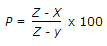[B].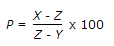[C].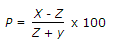[D].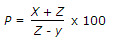[E].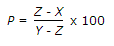Explanation:

No answer description available for this question.

 Shivam said: (Oct 16, 2014) How we got the answer?

 Ranjith said: (Jan 4, 2015) How to work to find answer?

 Nirav Bhatt said: (May 22, 2015) The fineness Modulus (FM) of Coarse aggregate is higher than combined and fine aggregate. The FM of fine aggregates is lowest. And FM of combined aggregate is in between. Here, X = Coarse aggregate = Largest value. Y = Fine aggregate = Lowest value. Z = Combined aggregate = Intermediate value. Compare it with the answer. You will get the required information.

 Dimpi said: (Oct 3, 2015) Z- Combined Aggregate. (Both fine & Coarse), So value should be highest?

 Darshan H A said: (Jun 29, 2016) Question should be percentage of fine aggregate to coarse aggregate not combined aggregate.

 Nayandeep said: (Aug 22, 2016) B and E options are same if we take sign common.

 Faisal said: (Nov 30, 2016) According to me, It is option D.

 Rohit Negi said: (Jul 14, 2017) According to me, the Answer is D.

 Sheela said: (Jul 25, 2017) B is correct. I agree.

 Chetan said: (Aug 25, 2017) No, answer is correct, according to question. @Darshan H A 100%correct. Let FA/CA=m then the ratio of FA with combined aggregate is m/ (m+1). Now solve you get the desired answer.

 Garry said: (Dec 2, 2017) None of the above is correct. Correct is, (X-Z)/(X-Y).

 S.Bhakta said: (Jun 16, 2018) Answer is obviously D.

 Virender Paul said: (Jul 14, 2018) Answer is correct. Because coaser particle will be greater than combined or finer so answer should be coarser-combined/combinef-fine.

 Manohar said: (Nov 20, 2018) pY+X(1-p)=1 * Z. It is p = (X-Z) ÷ (X-Y).

 Vivek Mishra said: (Feb 27, 2019) You are right, thanks @Manohar.

 Prasenjit said: (Mar 4, 2019) (CALCULATING RATIO OF FINE AGGREGATE TO COARSE AGGREGATE). Let "x1" is the percentage of fine aggregate to be combined with coarse aggregate. x can be calculated using the formula given below. x = [(F2-F)/(F-F1)] * 100. Where, F1 = Fineness modulus value of the fine aggregate. F2 = Fineness modulus value of coarse aggregate. F = Fineness modulus value of the combined aggregate. After finding the percentage of fine aggregate to be combined with the coarse aggregate by the above formula, their proportion can be calculated.

 Rishabh said: (Sep 26, 2020) It should be p={(X-Z)/(X-Y)}*100%.

 Pratusha Shankar Arepelli said: (Jun 3, 2021) How to calculate it? Please explain in detail.